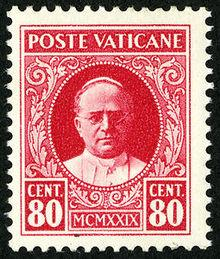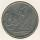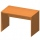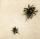# One hundred stamps

A hundred letter stamps cost a hundred crowns. Its costs are four levels - twenty tenths , one crown, two-crown and five-crown. How many are each type of stamps? How many does the problem have solutions?

n =  66

### Step-by-step explanation:

x1= 0.2*10 +1*85+2*4+5*1 = 100
x2= 0.2*15 +1*76+2*8+5*1 = 100
x3= 0.2*15 +1*79+2*4+5*2 = 100
x4= 0.2*20 +1*67+2*12+5*1 = 100
x5= 0.2*20 +1*70+2*8+5*2 = 100
x6= 0.2*20 +1*73+2*4+5*3 = 100
x7= 0.2*25 +1*58+2*16+5*1 = 100
x8= 0.2*25 +1*61+2*12+5*2 = 100
x9= 0.2*25 +1*64+2*8+5*3 = 100
x10= 0.2*25 +1*67+2*4+5*4 = 100
x11= 0.2*30 +1*49+2*20+5*1 = 100
x12= 0.2*30 +1*52+2*16+5*2 = 100
x13= 0.2*30 +1*55+2*12+5*3 = 100
x14= 0.2*30 +1*58+2*8+5*4 = 100
x15= 0.2*30 +1*61+2*4+5*5 = 100
x16= 0.2*35 +1*40+2*24+5*1 = 100
x17= 0.2*35 +1*43+2*20+5*2 = 100
x18= 0.2*35 +1*46+2*16+5*3 = 100
x19= 0.2*35 +1*49+2*12+5*4 = 100
x20= 0.2*35 +1*52+2*8+5*5 = 100
x21= 0.2*35 +1*55+2*4+5*6 = 100
x22= 0.2*40 +1*34+2*24+5*2 = 100
x23= 0.2*40 +1*37+2*20+5*3 = 100
x24= 0.2*40 +1*40+2*16+5*4 = 100
x25= 0.2*40 +1*43+2*12+5*5 = 100
x26= 0.2*40 +1*46+2*8+5*6 = 100
x27= 0.2*40 +1*49+2*4+5*7 = 100
x28= 0.2*45 +1*28+2*24+5*3 = 100
x29= 0.2*45 +1*31+2*20+5*4 = 100
x30= 0.2*45 +1*34+2*16+5*5 = 100
x31= 0.2*45 +1*37+2*12+5*6 = 100
x32= 0.2*45 +1*40+2*8+5*7 = 100
x33= 0.2*45 +1*43+2*4+5*8 = 100
x34= 0.2*50 +1*22+2*24+5*4 = 100
x35= 0.2*50 +1*25+2*20+5*5 = 100
x36= 0.2*50 +1*28+2*16+5*6 = 100
x37= 0.2*50 +1*31+2*12+5*7 = 100
x38= 0.2*50 +1*34+2*8+5*8 = 100
x39= 0.2*50 +1*37+2*4+5*9 = 100
x40= 0.2*55 +1*16+2*24+5*5 = 100
x41= 0.2*55 +1*19+2*20+5*6 = 100
x42= 0.2*55 +1*22+2*16+5*7 = 100
x43= 0.2*55 +1*25+2*12+5*8 = 100
x44= 0.2*55 +1*28+2*8+5*9 = 100
x45= 0.2*55 +1*31+2*4+5*10 = 100
x46= 0.2*60 +1*10+2*24+5*6 = 100
x47= 0.2*60 +1*13+2*20+5*7 = 100
x48= 0.2*60 +1*16+2*16+5*8 = 100
x49= 0.2*60 +1*19+2*12+5*9 = 100
x50= 0.2*60 +1*22+2*8+5*10 = 100
x51= 0.2*60 +1*25+2*4+5*11 = 100
x52= 0.2*65 +1*4+2*24+5*7 = 100
x53= 0.2*65 +1*7+2*20+5*8 = 100
x54= 0.2*65 +1*10+2*16+5*9 = 100
x55= 0.2*65 +1*13+2*12+5*10 = 100
x56= 0.2*65 +1*16+2*8+5*11 = 100
x57= 0.2*65 +1*19+2*4+5*12 = 100
x58= 0.2*70 +1*1+2*20+5*9 = 100
x59= 0.2*70 +1*4+2*16+5*10 = 100
x60= 0.2*70 +1*7+2*12+5*11 = 100
x61= 0.2*70 +1*10+2*8+5*12 = 100
x62= 0.2*70 +1*13+2*4+5*13 = 100
x63= 0.2*75 +1*1+2*12+5*12 = 100
x64= 0.2*75 +1*4+2*8+5*13 = 100
x65= 0.2*75 +1*7+2*4+5*14 = 100
x66= 0.2*80 +1*1+2*4+5*15 = 100Did you find an error or inaccuracy? Feel free to write us. Thank you!Tips to related online calculators
Do you solve Diofant problems and looking for a calculator of Diofant integer equations?

## Related math problems and questions:

• Czech crownsOldrich has one crown. Peter has five crowns coin, a two crown coin and a one-crown coin. Radek has twenty-crown banknote, ten banknote and five-crown coin. The boys got one fifty-one crown and one crown coin. How can they share the money fairly when they
• CrownSiblings collect a 2-crowns and 5-crowns. Together have 80 coins with a total of value 310 crowns. How many saved two-crowns and how many five-crowns?
• Crown coinsJana saves two-crown and five-crown coins. She has ten coins in the cashier. How many two crowns and how many five crowns shw have if she saved 29 crowns?
• Collection of stampsJano, Rado, and Fero have created a collection of stamps in a ratio of 5: 6: 9. Two of them had 429 stamps together. How many stamps did their shared collection have?
• Diofant equationIn the set of integers (Z) solve the equation: 212x +316y =0 Write result with integer parameter t in Z (parameter t = ...-2,-1,0,1,2,3... if equation has infinitely many solutions)
• Each chairEach chair costs twice less than two tables. One table costs 720 Czech crowns. How many crowns are the table and four chairs?
• Year 2018The product of the three positive numbers is 2018. What are the numbers?
• BasementsIn the first basement is more flies than the spiders, the second vice versa. Each basement had spiders and flies together 100 feet. Determine how many could be flies and spiders in the first and second basement. PS. We only need, when you write how many s
• Six-digit primesFind all six-digit prime numbers that contain each one of digits 1,2,4,5,7, and 8 just once. How many are they?
• The HotelThe Holiday Hotel has the same number of rooms on each floor. Rooms are numbered with natural numerals sequentially from the first floor, no number is omitted, and each room has a different number. Three tourists arrived at the hotel. The first one was in
• SchoolLess than 500 pupils attend school. When it is sorted into pairs, one pupil remains. Similarly, when sorted into 3, 4, 5 and 6 members team one remains. Sorted to seven members teams, no left behind. How many pupils are attending this school?
• Twenty-fiveHow many are three-digit natural numbers divisible by 25?
• Diofant equation250x + 120y = 5640
• Diofant 2Is equation 70x +52y = 34 solvable on the set of integers Z?
• TogetherIf 8 men, 10 women, 16 children collects ₹1024 in 4 days, how many days will be required for 6 men, 5 women and 4 boys to collect ₹768? (₹ is Indian Rupee)
• Sales of productsFor 80 pieces of two quality products a total sales is 175 Eur. If the first quality product was sold for n EUR per piece (n natural number) and the second quality product after 2 EUR per piece. How many pieces of the first quality were sold?
• Reminder and quotientThere are given the number C = 281, D = 201. Find the highest natural number S so that the C:S, D:S are with the remainder of 1,